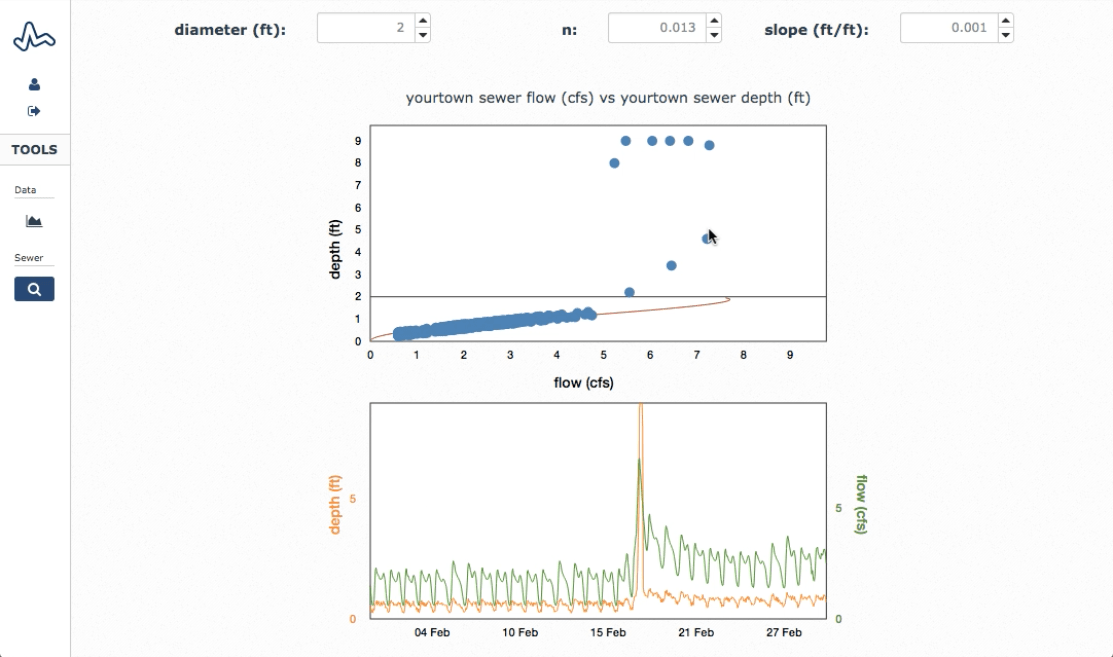Select PageA scatter plot is a tool used with area-velocity flow meters for system diagnostics. An area-velocity meter is an open channel flow meter that measures flow by making two separate measurements of depth and velocity. The depth is converted to cross sectional area using the geometry of the pipe or channel. The flow is then computed using the continuity equation by multiplying the flow area times the velocity, hence the name “A-V meter.”

#### Flow vs. Depth

A common way to evaluate the data collected by an A-V meter is to make a scatter plot of the flow versus the depth, as shown in the figure below from H2Ometrics. The scatter plot depicts the flow measurement along the x-axis and the depth measurement along the y-axis. The plot also depicts the diameter of the pipe as a horizontal line (black line in the plot below) and the flow-depth curve derived from the Manning’s equation (the red curve in the plot below).

#### Manning’s Curve

The Manning’s curve represents how the depth and flow relate under “normal” or “uniform” flow conditions in the pipe – that is the flow condition in which there are no obstructions, backwater effects or other unusual flow conditions in the pipe. This Manning’s line is a good reference to compare the meter data against to check the system performance. The Manning’s line is computed from the pipe diameter, slope and Manning’s “n” roughness value for the pipe.

#### Diagnosing Sewer Issues

When the scatter plot deviates from the Manning’s line, it indicates that the system is operating in a flow condition outside of “normal” or “uniform” flow. This can use useful for identifying and diagnosing sewer performance issues. For example, in the plot below, there is a large storm event that caused backwater conditions in the sewer. The backwater conditions are the points near the top of the plot when the depth was in the 8 to 9-foot range. This may indicate some downstream obstruction or capacity issue in the sewer system.

ADS has a nice series of scatter plot examples that walk through several different scatter plots types and what each one might be indicating in the sewer system. These can be helpful for diagnosing sewer issues from scatter plot data. You can even contact them and they will send you a free poster of the scatter plots.

#### Scatter Plots in H2Ometrics

The H2Ometrics scatter plot tool is interactive and dynamic. You can quickly plot different periods of data, and hover over recordings in either the scatter plot or the time-series plot and see the corresponding point in the other plot. This allows you to quickly explore the portions of the data that are driving the responses in the scatter plot, making it quick and easy to identify issues and diagnose problems.

If you would like to test out the scatter plot tools in H2Ometrics, you can use the “yourtown” sample data sets (flow and depth) in your account. Here is a link to a tutorial video on how to use the H2Ometrics scatter plots to get you started.